# Excel UNICODE FunctionArticle byExcelMojo Team## What Is UNICODE Function In Excel?

The Excel UNICODE function returns the numeric code of the selected characters or alphabets value. If the selected text is an alphabet, phrase, or sentence, it will return the code of the first alphabet or character, ignoring the other letters or words.

The UNICODE function in Excel is an inbuilt Text function, so we can insert the formula from the Function Library or enter it directly in the worksheet.

For example, the below table contains a list of text strings, and we must determine the code point of the first character in each string using the UNICODE function.

Select cell B2, enter the formula =UNICODE(A2), press the “Enter” key, and drag the formula from B2:B7 using the excel fill handle.

The output is shown above.

[Output Observation: The formula in each target cell accepts the excel cell reference to the corresponding text string, and returns the UNICODE value of the specified text.

Thus, the function UNICODE in Excel cell range B2:B7 returns the code points of the characters “T”, “η”, “★”, “9”, “[“, and “ý” in decimal notation.]

###### Key Takeaways
• The Excel UNICODE function checks the first character in the specified text to return the character’s code point in decimal notation. Users can use the function to determine a text’s UNICODE value.
• We can get the numeric code in different encoding formats, such as UTF-8 and UTF-16.
• We can apply the function with other inbuilt Excel functions, such as IF, SUBSTITUTE, RIGHT, and LEFT.
• Ensure to supply the given text in double quotations or the cell reference to the text as the argument value.

### UNICODE() Excel Formula

The syntax of the UNICODE Excel formula is,

The argument of the UNICODE Excel formula is,

• text: The text or string to find the UNICODE. It is a mandatory argument.

### How To Use UNICODE In Excel?

We can use the Excel UNICODE function in 2 ways, namely,

1. Access from the Excel ribbon.
2. Enter in the worksheet manually.

#### Method #1 – Access from the Excel Ribbon

Choose the target cell for output → select the “Formulas” tab → go to the “Function Library” group → click the “Text” option drop-down → select the “UNICODE” function, as shown below.

The Function Arguments window appears. Enter the argument in the Text field, and click OK, as shown below.

#### Method #2 – Enter in the Worksheet Manually

1. Select the target cell for the output.
2. Type =UNICODE( in the cell. [Alternatively, type =U or =UNICO and double-click the UNICODE function from the Excel suggestions.]
3. Enter the argument as a cell value or reference and close the brackets.
4. Press Enter to execute and get the required UNICODE value.

We will get the code point of the first character in each text using Excel UNICODE function.

The below table shows texts which are strings and symbols.

The steps to find the numeric codes using the UNICODE function are,

1. Select cell C3, and enter the formula =UNICODE(B3).

[Note: We can also enter the text directly as the argument value but in double quotations as =UNICODE(“σ is the symbol of standard deviation”)]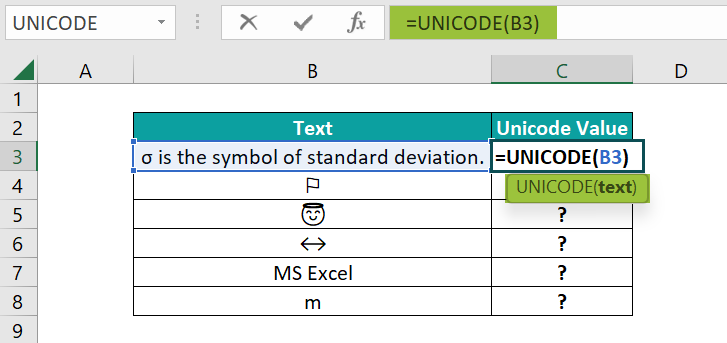Or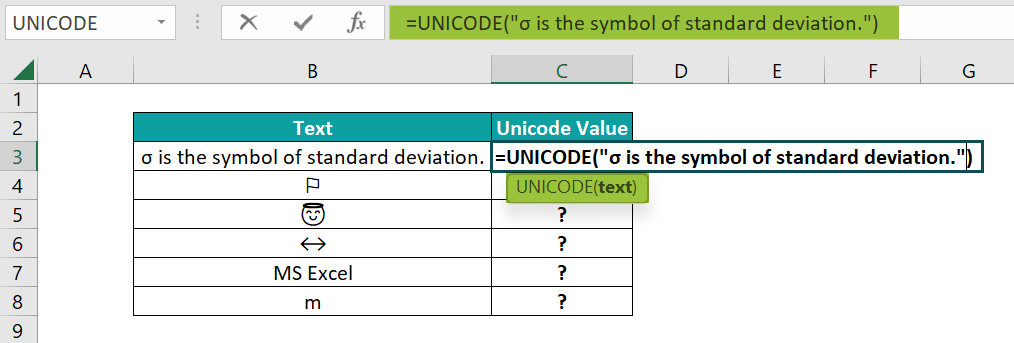2. Press Enter to execute the function.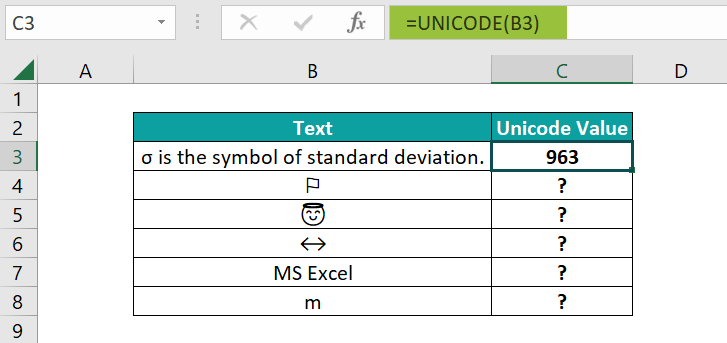[Alternatively, select the target cell C3 and insert the Excel UNICODE function using the path FormulasTextUNICODE to apply the function in the chosen cell.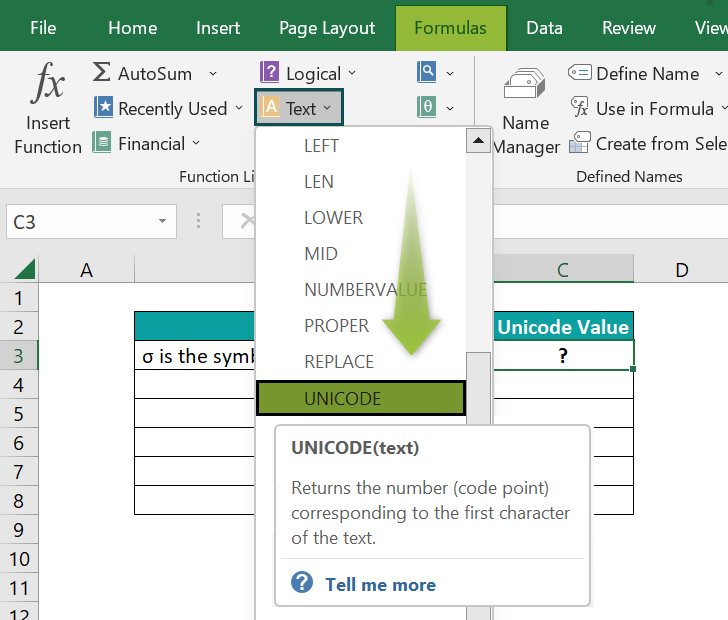The Function Arguments window appears. Enter the given cell reference in the Text field, and click “OK” to see the results.]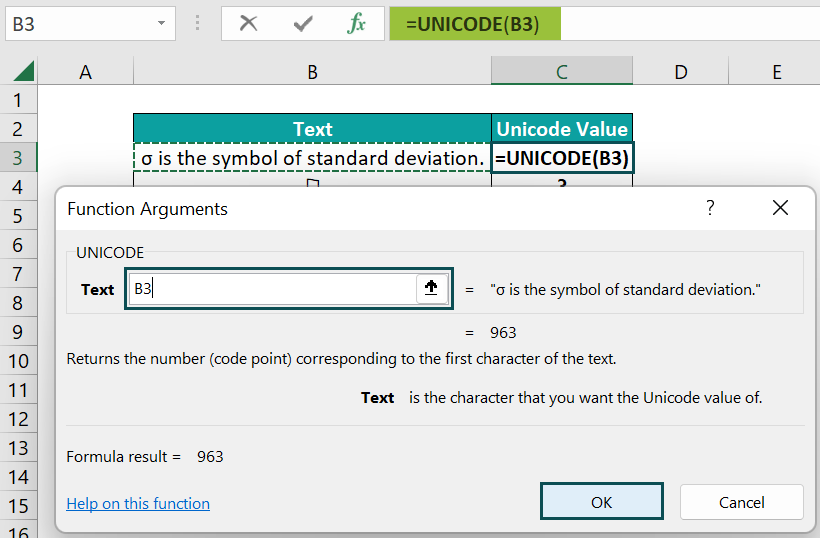3. Use the fill handle to drag the formula from cell C3 to C8. The output is shown below.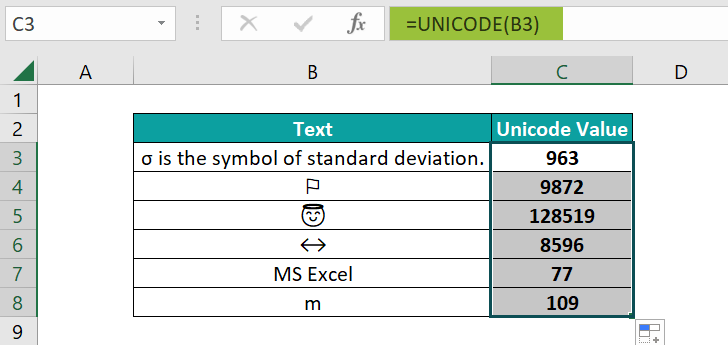[Output Observation: Let us consider cell C8 expression to test the formula. The UNICODE() accepts the cell reference to the text “m” as the argument value. And as the specified text contains a single character, the UNICODE function returns the code point of the letter “m”, 109, as the output.
On the other hand, the given texts in rows 3 and 7 are strings. So, the UNICODE() output in the corresponding target cells are the code points of the first characters, the symbol ‘σ ‘, and the letter “M”.

Also, the above example shows that the UNICODE() is case-sensitive. The function returns different code points for the same character in upper and lower case.]

### Examples

We will understand some advanced scenarios using the UNICODE in Excel examples.

#### Example #1

We will determine the UNICODE value of each enclosed alphanumeric symbol for the below table with a grocery list. We have used enclosed alphanumeric symbols (a number enclosed in a circle) while numbering the items in the list in the worksheet.

The steps to find the code using the UNICODE formula are,

• Step 1: Select the target cell C3, enter the formula =UNICODE(B3), and press Enter.
• Step 2: Using the fill handle, drag the formula from cell C3 to C7. The output is shown below.

[Output Observation: Let us see the cell C7 expression to understand how the UNICODE() works. The function accepts the text “ All Purpose Flour”as the input string. And as the enclosed alphanumeric value “” is the first character in the specified text, the function returns its code point, 9316.]

#### Example #2

We can obtain UNICODE in various encodings, such as UTF-8 and UTF-16, with the UTF-8 being the most-used encoding format on the web.

Moreover, the first 128 code points achieved using the Excel UNICODE function for the UTF-8-character set are for the ASCII characters.

We shall now see how to use the function in the Excel UNICODE UTF-8 scenario.

Column B contains a list of ASCII (rows 2 to 5) and non-ASCII (rows 6 to 8) characters.

The steps to determine the Excel UNICODE UTF-8 values are as follows:

• Step 1: Select the target cell C2, enter the formula =UNICODE(B2), and press Enter.
• Step 2: Using the fill handle, drag the formula from cell C2 to C8. The output is shown below.

[Output Observation: In the target cells C2:C5, the UNICODE() returns the ASCII codes of the characters “a”, “b”, “c”, and “d”, respectively, as the required UNICODE values in decimal notation.

On the other hand, the first character in each text in cells B6:B8 is the same non-ASCII character, the Hiragana Letter A. So, the UNICODE() output is the same in the corresponding target cells, 12354.]

#### Example #3

We will use the Excel UNICODE function with the IF(), LEFT(), and SUBSTITUTE() Excel functions.

The following table contains five steps to evolving as a financial advisor, with each step represented as a roman numeral. And we must display the steps without the roman numerals in column B.

The steps to obtain the desired outcome are,

• Step 1: Select the target cell B2, enter the below formula, and press Enter.

=IF(UNICODE(LEFT(A2))>=8544,IF(UNICODE(LEFT(A2))<=8584,SUBSTITUTE(A2,LEFT(A2),””)),A2)

• Step 2: Use the fill handle to drag the formula from cell B2 to B6. The output is shown below.

[Output Observation: Let us see the cell B6 expression to check how the formula works.

All the LEFT excel function instances return the leftmost character of the cell A6 text, , the roman numeral.

The first UNICODE() is the outer IF condition, and it returns the code point of the roman numeral , 8548. And as the code point is more than 8544, the IF condition holds in cell B6. So, the inner IF excel function executes. The second UNICODE() works the same way as explained above and returns the UNICODE value, 8548, a value that is less than 8584. So, the SUBSTITUTE excel function executes. The function substitutes the LEFT() output, the roman numeral , in the original text with a space. And thus, the final result is a text without the roman numeral.]

### Important Things To Note

• In the case of the given text having partial surrogates or invalid data types, the Excel UNICODE function throws the #VALUE! error.
• For UNICODE values falling outside the acceptable range, the UNICODE() returns the #VALUE! error.
• Suppose we supply the given text directly as the UNICODE() argument but without enclosing the text in double quotations. Then, the function returns the #NAME? error.

1. Where is the UNICODE function in Excel?

The UNICODE function is in the Formulas tab. Navigate the path FormulasFunction LibraryTextUNICODE to apply the function in the chosen cell.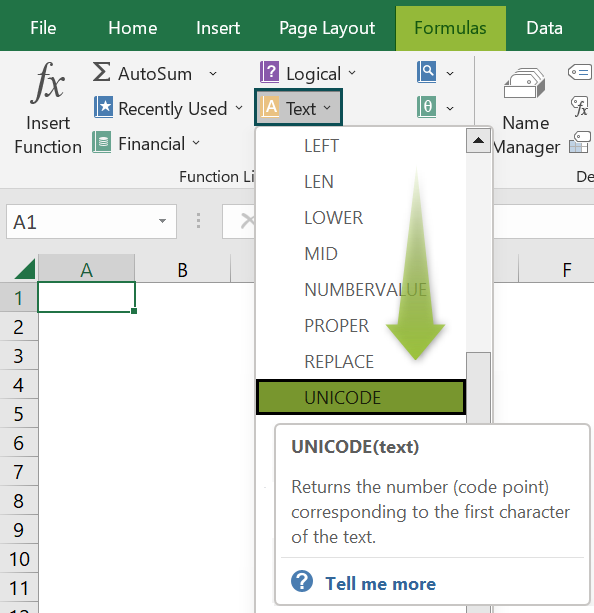2. How to apply the UNICODE function in Excel VBA?

We can apply the UNICODE function in Excel VBA using the below method:

Application.WorksheetFunction.Unicode(Arg1)

The following table shows a list of texts. We will find the UNICODE values for the given texts.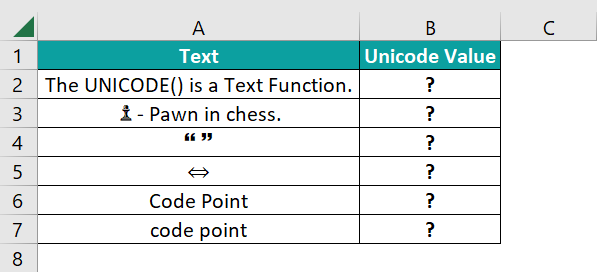The steps to apply the UNICODE function in Excel VBA are,

• Step 1: With the active worksheet containing the above table open, access the VBA Editor using the shortcut keys Alt + F11.• Step 2: Choose the required VBAProject, and navigate the path InsertModule to access a new module.• Step 3: Enter the below given VBA code in the Module1 window to execute the UNICODE function in the required cells.

Sub Unicode_fn()
Dim ws As Worksheet
Set ws = Worksheets(“UNICODE_FAQ”)
ws.Range(“B2”) = Application.WorksheetFunction.Unicode(ws.Range(“A2”))
ws.Range(“B3”) = Application.WorksheetFunction.Unicode(ws.Range(“A3”))
ws.Range(“B4”) = Application.WorksheetFunction.Unicode(ws.Range(“A4”))
ws.Range(“B5”) = Application.WorksheetFunction.Unicode(ws.Range(“A5”))
ws.Range(“B6”) = Application.WorksheetFunction.Unicode(ws.Range(“A6”))
ws.Range(“B7”) = Application.WorksheetFunction.Unicode(ws.Range(“A7”))
End Sub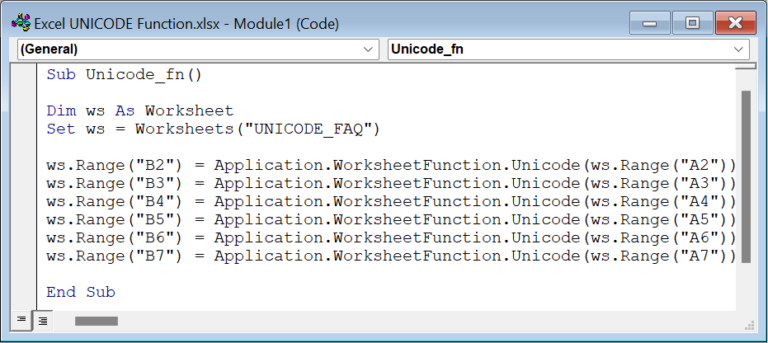• Step 4: Click on the Run Sub/UserForm icon in the menu to run the entered code.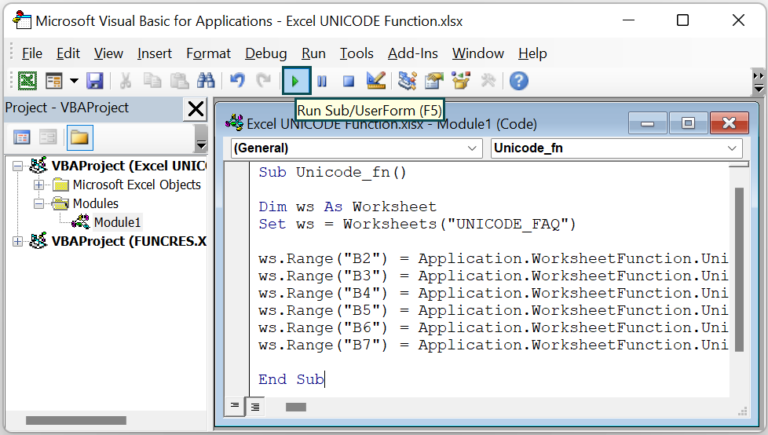Finally, we can open the active worksheet to view the output in the target cells.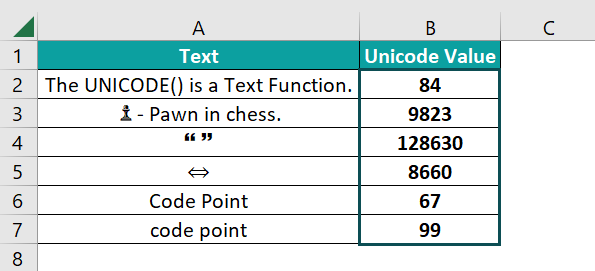Thus, the function UNICODE() in the cell range B2:B7 returns the code points of the characters “T”, “”, “🙶 ”, “”, “C“, and “c” in decimal notation.

3. When does the UNICODE function in Excel throw errors?

The UNICODE function returns errors in the following scenarios:
We supply the text directly as the text argument but without enclosing the value in double quotations.
The specified text includes partial surrogates or invalid data formats.
• UNICODE values lie outside the allowed range.